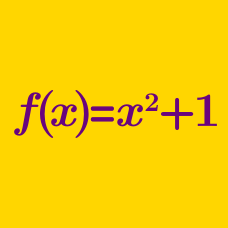Algebra

# Symbolic Operators

For positive integer $k$, let $\bullet k\bullet = k^{2}-(k-1)^{2}$. What is the value of $\bullet 7\bullet$?

(A) $\ \ -36$
(B) $\ \ 7$
(C) $\ \ 13$
(D) $\ \ 49$
(E) $\ \ 85$

For positive integers $m$ and $n$, let $m \nabla n$ be defined as $m \nabla n = (\frac{m}{n})^{-1} - (\frac{n}{m})^{-1}$. What is the value of $2 \nabla 15$?

(A) $\ \ -\frac{15}{2}$

(B) $\ \ -\frac{221}{30}$

(C) $\ \ \frac{221}{30}$

(D) $\ \ \frac{2}{15}$

(E) $\ \ \frac{229}{30}$

For positive integers $m$, $k$, and $n$, let $m\breve{k}n$ be defined as $m\breve{k}n = k\frac{m}{n}$, where $k\frac{m}{n}$ is a mixed fraction. What is the value of $6\breve{4}1 + 1\breve{4}6$?

(A) $\ \ 0$

(B) $\ \ 5$

(C) $\ \ \frac{85}{6}$

(D) $\ \ 700$

(E) $\ \ 787$

For positive integers, $x$, $y$, and $z$, let $\widehat{x,y,z}$ be defined by $\widehat{x,y,z} = zx - yz - xy$. What is the value of $m$ if $\widehat{10,m,10} = \widehat{7,3,1}?$

(A) $\ \ -\frac{631}{20}$

(B) $\ \ -\frac{117}{20}$

(C) $\ \ 0$

(D) $\ \ \frac{21}{100}$

(E) $\ \ \frac{117}{20}$

For a positive integer $n$, let $\fbox{n} = 1-2+3-4 \ldots n$. For example, $\fbox{5}=1-2+3-4+5=3.$ Which of the following is equal to $\fbox{6}-\fbox{48}$?

(A) $\ \ -1155$
(B) $\ \ -27$
(C) $\ \ -21$
(D) $\ \ -\fbox{6}$
(E) $\ \ 21$

×